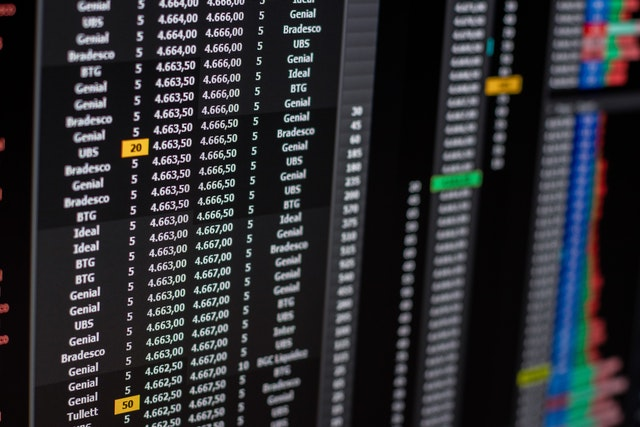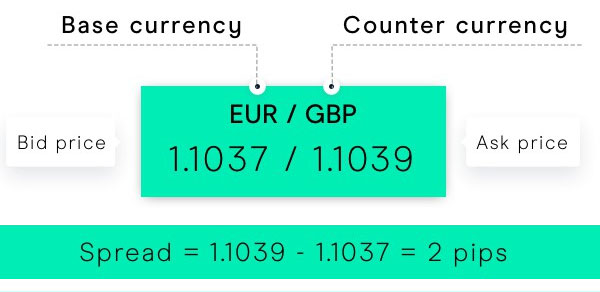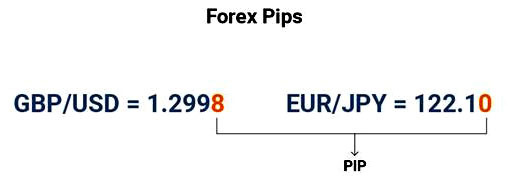# Understanding and How to Calculate Pips in Trading, Traders Must Know!

The pip value is commonly used by traders to calculate the range of price changes between two currencies in a transaction. Although it is often used, in fact some people are still not familiar with what pip is in forex and crypto trading. To find out the definition and how to calculate pips in trading, see the full information in the article below.pixels.com/Romulo Queiroz

## What is a Pip in Trading?

In general, pip in trading stands for “percentage in point” or “price interest point”.

The IG Markets team defines a pip as a measure of the movement in value between two currencies in a trade.(century.eu)

Meanwhile, the meaning of pips in trading according to Adam Hayes of Investopedia is the smallest price movement step that can be changed by market conventions.

John Russell of The Balance reveals that pips are often used to calculate the rates that participants in the forex market pay when trading currencies.

Adam adds that the pip value is also often used by traders to calculate the spread (range of values) between the bid (bid) and ask (ask) prices of a currency pair. The pip value is also useful for estimating the amount of profit or loss experienced by a trader during trading.

During trading, the pip value is used by traders to identify stop losses that can limit potential losses. According to the CMC Markets team, pip has helped traders to calculate the most appropriate position size to ensure that they are not taking too much risk by opening too large a trade position.

## How to Calculate Pips in Trading

In financial asset trading, the smallest price change occurs at the last decimal place of the asset price. The IG Markets team explains that the difference in pip values ​​is determined by the currency pair being traded, the trade size and the exchange rate of the currency pair.(dailyfx.com)

The CMC Markets team explains that pip values ​​for most major currencies such as USD, EUR and GBP are priced around 1/100th of 1%, or up to four decimal places (0.0001).

In comparison, the pip value of a currency pair using the Japanese yen (JPY) is priced at 1% or two decimal places. In this context, one pip represents an asset's price movement of 0.01. For example, if GBP/JPY moves from 150.00 to 150.04, the price has moved four pips.

Reporting from CMC Markets, here is how to calculate the pip value in trading.

Pip value (general) = (0.0001 x trade value) / spot price

Meanwhile, how to calculate the pip value in trading for currency pairs using the Japanese yen is as follows:

Pip value (JPY) = (0.01 x trading value) / spot price

## Then, 1 Pip How Much Dollar?

Cory Mitchell of The Balance explains that the type of currency used to open a trading account will determine the pip value of various currency pairs. Users who open an account with funds in US dollars, the pip value will always be constant as follows.

The pip values above apply to any pair, provided that USD is listed second as the counter currency or ask price. For example, euro/US dollar (EUR/USD), British pound/US dollar (GBP/USD), Australian dollar/US dollar (AUD/USD), and New Zealand dollar/US dollar (NZD/USD).

When USD is not listed as second, the pip value calculation will change. If USD is the base currency or the bid price, the pip value is calculated by dividing the lot price by the exchange rate.

Examples are as follows. If the US dollar and Canadian dollar (USD/CAD) exchange rate is 1.33119, the pip value for the standard lot is \$7.51. This value is obtained by dividing the lot price and the exchange rate, which is \$10 /1.3319 = \$7.51

That was information about understanding and how to calculate pips in trading. Not too difficult, right? Pip itself applies in the forex and crypto markets.

No Comment